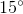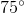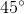# AP Physics C: Mechanics : Understanding Distances

## Example Questions

### Example Question #5 : Mechanics Exam

An object starts from rest and reaches a velocity of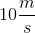after accelerating at a constant rate for four seconds. What is the distance traveled in this time?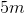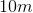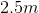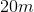Explanation:

Since the acceleration is constant in this problem, we can apply the kinematics given equation to calculate the distance: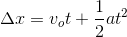First, we need to calculate the acceleration.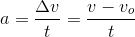Plug in our velocity and time values to find the acceleration.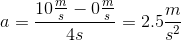Now we can return to the kinematics equation and solve for the distance traveled: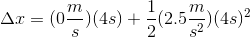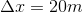### Example Question #6 : Mechanics Exam

At the moment a car is passed by another car constantly traveling at, it begins to accelerate at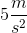. In how many seconds does this car catch up to and pass the other car?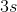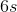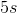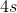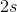Explanation:

First, find the displacement equations for both cars. Car 1 will be the car that is initially stationary; car 2 will be the car traveling with constant velocity.

Car 1: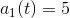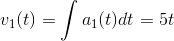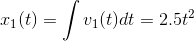Car 2: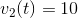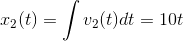Now, set those displacement equations equal to each other and solve for.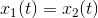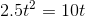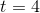The accelerating car will catch and pass the car traveling at constant velocity after 4 seconds.

### Example Question #1 : Mechanics Exam

An object moving along a line has a displacement equation of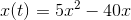, whereis in seconds. For what value ofis the object stationary?Explanation:

Use the fact that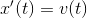to find the velocity equation, and then solve forwhen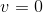.Take the derivative of the displacement equation.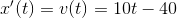Set the velocity equal to zero.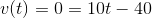Solve for the time.### Example Question #8 : Mechanics Exam

An object is moving on a line and has displacement equation of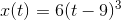, whereis in seconds. At what value ofis the object not accelerating?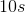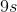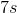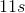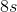Explanation:

Take the second derivative of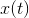, to find the acceleration function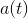. Then find the value ofwhere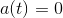. The fact that it is simplyand not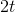orinside that quantity means that using the Chain Rule becomes decidedly easier.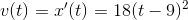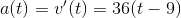Set the acceleration fuction equal to zero and solve for the time.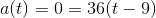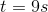### Example Question #1 : Linear Motion

A projectile is launched out of a cannon or launch tube with zero air resistance or friction. At what angle (in degrees) should the projectile be launched to maximize the distance it travels?